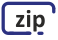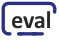Basic Information
Lecturers: Gerhard Schmidt (lecture), Karolin Krüger (exercise)
Room: Building F, room SR-IV
E-mail: This email address is being protected from spambots. You need JavaScript enabled to view it.
Language: English
Target group: Students in electrical engineering and computer engineering
Prerequisites: Basics in system theory
Contents:

Students attending this lecture should learn the basics of adaptive filters. To achieve this, necessary algorithms will be derived and applied to problems arising in speech and audio processing. The algorithms comprise Wiener filtering, linear prediction, and adaptive schemes such as the NLMS algorithm, affine projection, and the RLS algorithm. For applications from speech and audio processing we use noise and reverberation reduction, echo cancellation, and beamforming.

Topic overview:

• Introduction and application examples
• Signal properties and cost functions
• Wiener filter and principle of orthogonality
• Linear prediction
• RLS algorithm
• LMS algorithm and its normalized version
• Affine projection algorithm
• Efficient processing structures
• Applications of linear prediction

No news yet.

#### Schedule

The following schedule regarding lectures and excercises is preliminary and may be adapted during the semester.

Each event starts at 8:15 h and might use the whole slot (lecture and exercise) until 11:45 h.

Date Event
12.04.2023 Lecture: Introduction
19.04.2023 Lecture: Wiener filter
26.05.2023 Lecture: Linear prediction
03.05.2023 Exercise: Wiener Filter and linear prediction
10.05.2023 Lecture: Algorithms part I
17.05.2023 Lecture: Algorithms part II
24.05.2023 Lecture: Control
31.05.2023 Exercise: Algorithms and Control
07.06.2023 Lecture: Processing structures
14.06.2023 Lecture: Applications of linear prediction
21.06.2023 Student talks
28.06.2023 Exercise: Processing structures and questions

#### Lecture SlidesSlides of the lecture "Introduction"
(Introduction, boundary conditions of the lecture, applications)Slides of the lecture "Wiener Filter"
(basics, principle of orthogonality, suppression of background noise)Slides of the lecture "Linear Prediction"
(derivation of linear prediction, Levinson-Durbin recursion)Slides of the lecture "Algorithms (Part 1 of 2)"
(RLS algorithm, LMS algorithm [part 1 of 2])Slides of the lecture "Algorithms (Part 2 of 2)"
(LMS algorithm [part 2 of 2], affine projection algorithm)Slides of the lecture "Control"
(basic aspect, pseudo-optimal control parameters)Slides of the lecture "Processing Structures"
(polyphase filterbanks, prototype lowpass filter design)Slides of the lecture "Applications of Linear Prediction"
(Improving the speed of convergence, filter design)

#### ExtensionsExtension for the lecture "Wiener Filter"
(derivation of the error surface)

#### Matlab DemosMatlab demo (GUI based) for adaptive system identificationMatlab demo (GUI based) for adaptive noise suppressionMatlab demo (GUI based) for linear predictionMatlab demo (GUI based) for the NLMS algorithmsMatlab demo (GUI based) for prediction-based filter design

#### Exercises

We provide additional videos and corresponding materials (e.g., questions and answers) for each topic below.

Exercises will be conducted according to the (preliminary) schedule above. Please register for the corresponding OLAT course to receive further information by mail (https://lms.uni-kiel.de/url/BusinessGroup/3276832778). Please bring a notebook for the exercises.

Video Content Material

Wiener filter:

• summary
• comprehension questions
• python demoLinear prediction:

• summary
• comprehension questions
• signal visualization
• python demoAlgorithms:

• summary
• explaining algorithms
• comprehension questions
• python demoControl:

• motivation/summary
• comprehension questions
• python demoProcessing structures:

• summary
• comprehension questions
• python demo#### Student Talks

As part of the lecture, each student will give a talk about a certain topic as a prerequisite to sit the exam. The aim is both to give you the chance to work on an adaptive filter-related topic that interests you, and to improve your presentational skills. The talks should take ten minutes, plus 2.5 minutes of discussion and 2.5 minutes of feedback.

21.06.2023

Time Topic Presenter(s)
08:15 h Opening Gerhard Schmidt
08:20 h Adaptive Beamforming Patrick Nwobodo
08:35 h Adaptive Filters in Digital Transmission Johannes Diers
08:50 h Channel Equalization Maximilian Klein
09:05 h Adaptive Filters for Active Sonar Detection Lukas Schirmer
09:20 h Adaptive Filters in Economics and Finance Luca Lohmann
09:35 h Application of Adaptive Filters in the Medical Field Bamo SadiqCurrent evaluationCompleted evaluations